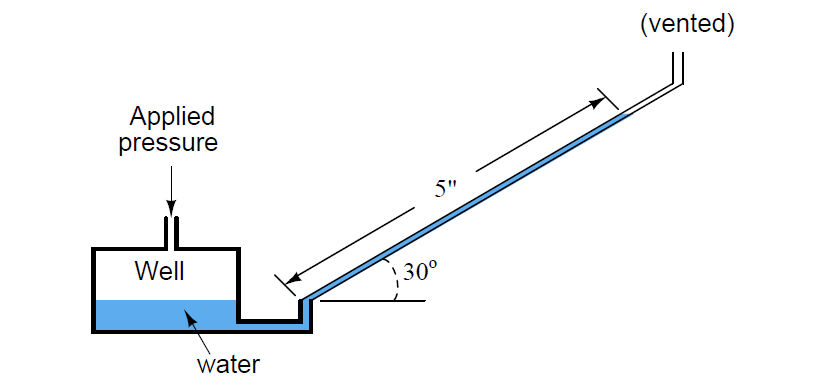# How much Pressure is applied to Inclined Water Manometer?

## Inclined Water Manometer

#### Question 1:

How much pressure, in inches of water column, is being applied to this inclined water manometer to displace water 5 inches along the length of the tube, inclined at an angle of 30o from horizontal? Assume a negligible change in liquid level inside the “well” throughout the measurement range of the instrument:Applied pressure = 2.5 ”W.C.

What matters in manometer calculations is the vertical height diﬀerence between the two liquid columns. Inclining one or more of the tubes only causes the liquid to displace further along the tube(s); it does not change the vertical height necessary to balance the applied pressure.

Thus, with a 30o inclined tube, a liquid displacement of 5 inches along the length of the tube equates to one-half that (sin 30o = 0.5). Therefore, the applied pressure is 2.5 inches of water column.

Note that the inclined manometer makes very sensitive pressure measurements possible using standarddensity liquids such as water! Great care, though, must be taken in ensuring the instrument is level (that the inclined tube is at precisely the angle it should be).

#### Question 2:

How much pressure, in inches of water column, is being applied to this inclined water manometer to create a total displacement of 14 inches along the length of the tubes, inclined at angles of 20o from horizontal?

Assume the base of this manometer is located 24 inches above ground level.Next, convert this pressure into units of kPa

Applied pressure = 1.193 kPa

#### More Questions:

1. Identify which fundamental principles of science, technology, and/or math apply to each step of your solution to this problem. In other words, be prepared to explain the reason(s) “why” for every step of your solution, rather than merely describing those steps.

2. Does inclining a manometer make it more or less sensitive to applied pressure? Develop a “thought experiment” where you could test a manometer to answer this question.

3. Is it possible to make a well version of an inclined manometer so that we need only to read one liquid column?

4. What will happen to a manometer if it is exposed to a gas pressure greater than its measurement range?

5. Would a micromanometer be more or less sensitive to applied pressure than this inclined manometer?

Credits: Tony R. Kuphaldt